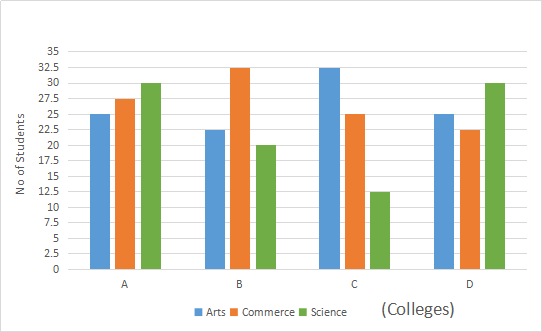## SBI Clerk Quant Test 1

Instructions

Study the following graph and answer the questions given below it.
Number of Students from Various Faculties studying in Various Colleges (Number in Thousands)Q 1

What is the respective ratio of the number of students from the faculty of Arts studying in college D?

Q 2

What is the average number of students studying in college A from all the faculties together?

Q 3

What is the average number of students from the faculty of Arts from all the colleges together?

Q 4

The students from the faculty of Commerce studying in college B are approximately what per cent of the total number of students studying in the same college?

Q 5

What is the difference between the total number of students from the faculty of commerce from all the colleges together and the total number of students from the faculty of Science from all the colleges together?